## ICSE Physics Previous Year Question Paper 2018 Solved for Class 10

General Instructions :

• Answers to this Paper must be written on the paper provided separately.
• You will not be allowed to write during the first 15 minutes.
• This time is to be spent in reading the Question Paper.
• The time given at the head of this Paper is the time allowed for writing the answers.
• Section I is compulsory. Attempt any four questions from Section II.
• The intended marks for questions or parts of questions are given in brackets [ ].

Section – I [40 Marks]
(Attempt all questions from this Section)

Question 1.
(a) (i) State and define the SI unit of power. 
(ii) How is the unit horse power related to the SI unit of power?
(b) State the energy changes in the following cases while in use: 
(i) An electric iron.
(ii) A ceiling fan.
(c) The diagram below shows a lever in use: 
(i) To which class of levers does it belong?
(ii) Without changing the dimensions of the lever, if the load is shifted towards the fulcrum what happens to the mechanical advantage of the lever?(d) (i) Why is the ratio of the velocities of light of wavelengths 4000 A and 8000 A in vacuum 1:1?
(ii) Which of the above wavelengths has a higher frequency? 
(e) (i) Why is the motion of a body moving with a constant speed around a circular path said to be accelerated?
(ii) Name the unit of physical quantity obtained by the formula 2K/V2 where K: kinetic energy, V: Linear velocity.
(a) (i) The SI unit of power is watt (W). The power of an agent is said to be one watt if it does one joule of work in one second.
(ii) 1 hp = 746 W

(b) (i) Electrical into heat energy
(ii) Electrical

(c) (i) Second class .
(ii)It increases

(d) (i) Because all wavelengths of light travel with the same velocity in vacuum
(ii) 4000 A

(e) (i) Because at every point of motion the direction of speed changes i.e., the body possesses velocity which changes with time.
(ii) J s -2 m -2

Question 2.
The power of a lens is -5D. 
(a) (i) Find its focal length.
(ii) Name the type of lens.
(b) State the position of the object in front of a converging lens if:
(i) It produces a real and same size image of the object.
(ii) It is used as a magnifying lens.
(c) (i) State the relation between the critical angle and the absolute refractive index of a medium.
(ii) Which colour of light has a higher critical angle? Red light or Green light. 
(d) (i) Define scattering. 
(ii) The smoke from a fire looks white. Which of the following statements is true?
1. Molecules of the smoke are bigger than the wavelength of light.
2. Molecules of the smoke are smaller than the wavelength of light.
(e) The following diagram shows a 60°, 30°, 90° glass prism of critical angle 42°. Copy the diagram and complete the path of incident ray AB emerging out of the prism marking the angle of incidence on each surface.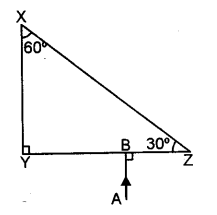(a) (i) f =$$\frac { 1 }{ P }$$ = $$\frac { 1 }{ -5 }$$ = -0.2 m = 20cm
(ii) Concave

(b) (i) 2F
(ii) Between F and 2F

(c) (i) n = $$\frac { 1 }{ sin C }$$
(ii) Red

(d) (i) Scattering is a general physical process where light is forced to deviate from a straight trajectory by one or more paths due to localized non-uniformities in the medium through which it passes
(ii) Molecule of the smoke are bigger than the wavelength of the light.

(e) The diagram is as shown.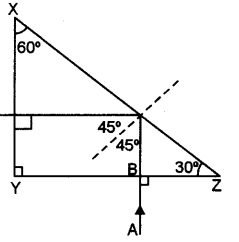Question 3.
(a) Displacement distance graph of the two sound waves A and B, travelling in a medium, are as shown in the diagram below.Study the two sound waves and compare their:
(i) Amplitudes
(ii) Wavelengths
(b) You have three resistors of values 2 Ω , 3 Ω and 5 Ω How will you join them so that the total resistance is more than 7 Ω? 
(i) Draw a diagram for the arrangement.
(ii) Calculate the equivalent resistance.
(c) (i) What do you understand by the term nuclear fusion? 
(ii) Nuclear power plants use nuclear fission reaction to produce electricity. What is the advantage ofproducing electricity by fusion reaction?
(d) (i) What do you understand by free vibrations of a body? 
(ii) Why does the amplitude of a vibrating body continuously decrease during damped vibrations?
(e) (i) How is the emf across primary and secondary coils of a transformer related with the number of turns of coil in them? 
(ii) On which type of current do transformers work?
(a) (i) AA/AB = 20/10 = 2
(ii) λB > λA

(b) (i) All of these can be connected in series as shown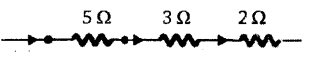(ii) R = 5 + 3 + 2 = 10 Ω

(c) (i) K is a process in which two or more smaller nuclei combine to form a bigger nucleus.
(ii) There is no pollution in nuclear fusion.

(e) (i) $$\frac{\mathrm{V}_{\mathrm{P}}}{\mathrm{V}_{\mathrm{S}}}$$ =  $$\frac{\mathrm{N}_{\mathrm{P}}}{\mathrm{N}_{\mathrm{S}}}$$
(ii) Alternating current

Question 4.
(a) (i) How can a temperature in degree Celsius be converted into SI unit of temperature? 
(ii) A liquid X has the maximum specific heat capacity and is used as a coolant in Car radiators. Name the liquid X.
(b) A solid metal weighing 150 g melts at its melting point of800 °C by providing heat at the rate of 100 W The time taken for it to completely melt at the same temperature is 4 mm. What is the specific latent heat of fusion of the metal? 
(c) Identify the following wires used in a household circuit: 
(i) The wire is also called as the phase wire.
(ii) The wire is connected to the top terminal of a three-pin socket.
(d) (i) What are isobars? 
(ii) Give one example of isobars.
(e) State any two advantages of electromagnets over permanent magnets. 
(a) (i) 1°C = 273 K
(ii) Water

(b) Using Q=mL, 100 x 4 x 60 x =150 x 10-3 x L or L =$$\frac{100 \times 4 \times 60}{150 \times 10^{-3}}$$ = L = 1.6 x 10J Kg-1

(c) (i) Live wire
(ii) Earth wire

(d) (i) Elements having same mass number but different atomic number.
(ii) 10S, 40Cl, 40Ar, 40K, and 40Ca.

(e) (i) Strength can be increased or decreased during working
(ii) Polarities can be changed during working.

Section – II [40 Marks]
(Attempt any FOUR Questions from this Section)

Question 5.
(a) (i) Derive a relationship between SI and C.G.S. unit of work. 
(ii) A force acts on a body and displaces it by a distance S in a direction at an angle θ with the direction of force. What should be the value of θ to get the maximum positive work?
(b) A half metre rod is pivoted at the centre with two weights of 20 gf and 12 gf suspended at a perpendicular distance of 6 cm and 10 cm from the pivot respectively as shown below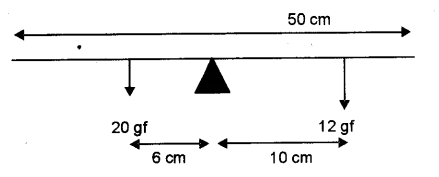(i) Which of the two forces acting on the rigid rod causes clockwise moment?
(ii) Is the rod in equilibrium?
(iii) The direction of 20 kgf force is reversed. What is the magnitude of the resultant moment of the forces on the rod?
(c) (i) Draw a diagram to show a block and tackle pulley system having a velocity ratio of 3 marking the direction of load(L), effort(E) and tension(T).
(ii) The pulley system drawn lifts a load of 150 N when an effort of 60 N is applied. Find its mechanical advantage.
(iii) Is the above pulley system an ideal machine or not? 
(a) (i) 1 J = 1 kg × 1 m2 × 1 s-2 = 103 g × 104 cm2 × 1 s-2 = 107 g cm2 s-2 = 107 erg
(ii) W = FS cos θ = FS (maximum) if cos θ = 1 or θ = 0°

(b) (i) 12 gf
(ii) yes
(iii) 120 +120 = 240 dyne cm

(c) (i) The diagram is as shown.(ii) Actual MA number of pulleys in the system = 3. Observed MA = $$\frac { load }{ effort }$$ = $$\frac { 150 }{ 60 }$$=2.5

(iii) Since Observed MA is lesser than the theoretical MA therefore, the machine is not an ideal machine.

Question 6.
(a) A ray of light XY passes through a right angled isosceles prism as shown below(i) What is the angle through which the incident ray deviates and emerges out of the prism?
(ii) Name the instrument where this action of prism is put into use.
(iii) Which prism surface will behave as a mirror?
(b) An object AB is placed between O and F1 on the principal axis of converging lens as shown in the diagram.Copy the diagram and by using three standard rays starting from point A, obtain an image of the object AB.
(c) An object is placed at a distance of 12 cm from a convex lens of focal length. 8 cm. Find: 
(i) the position of the image
(ii) nature of the image
(a) (i) 90°
(ii) Periscope
(iii) AB

(b) The figure is as shown.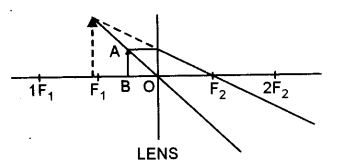Given u = – 12 cm,f= + 8 cm, v = ?, nature= ?
Using the lens formula $$\frac{1}{f}=\frac{1}{v}-\frac{1}{u}$$ we have
$$\frac{1}{f}=\frac{1}{v}-\frac{1}{u}$$ = $$\frac{1}{8}+\frac{1}{-12}$$ = $$\frac{3-2}{24}$$ = $$\frac{1}{24}$$
Or v = 24 cm, Image will be real and inverted.

Question 7.
(a) Draw the diagram of a right angled isosceles prism which is used erect. 
(b)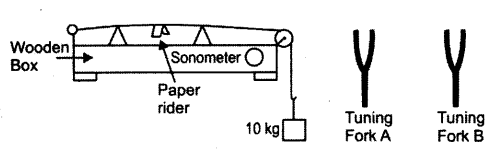The diagram above shows a wire stretched over a sonometer. Stems of two vibrating tuning forks A and B are touched to the wooden box of the sonometer. It is observed that the paper rider (a small piece of paper folded at the centre) present on the wire flies off when the stem of vibrating tuning fork B is touched to the wooden box but the paper just vibrates when the stem of vibrating tuning fork A is touched to the wooden box.
(i) Name the phenomenon when the paper rider just vibrates.
(ii) Name the phenomenon when the paper rider flies off.
(iii) Why does the paper rider fly off when the stem of tuning fork B is touched to the box?
(c) A person is standing at the sea shore. An observer on the ship, which is anchored in between a vertical cliff and the person on the shore, fires a gun. The person on the shore hears two sounds, 2 seconds and 3 seconds after seeing the smoke of the fired gun. If the speed of sound in the air is 320 m s-1 then calculate:
(i) the distance between the observer on the ship and the person on the shore.
(ii) the distance between the cliff and the observer on the ship.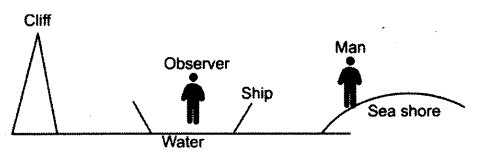(a) The diagram is as shown.(b) (i) Forced vibrations
(ii) Resonance
(iii) The wire of the sonometer begins to vibrate with its natural frequency. This is called resonance. At resonance the amplitude of vibration becomes very large.

(c) Let distance between the observer on the ship and person on the shore be x. For the first sound which the person hears after 2 second, we have
v= $$\frac { x }{ t }$$ => x = vt = 320 x 2 = 640 m
Let distance between the ship and the cliff be x, then in the second case
V = $$\frac{2 x+640}{t}$$ ⇒2x + 640 = vt = 320 × 3 = 960
or
2x = 960 – 640 = 320m
x= $$\frac{320}{2}$$ = 160 m

Question 8.
(a) (i) A fuse is rated 8 A. Can it be used with an electrical appliance rated 5 kW, 200 V? Give a reason. 
(ii) Name two safety devices which are connected to the live wire of a household electric circuit.
(b) (i) Find the equivalent resistance between A and B.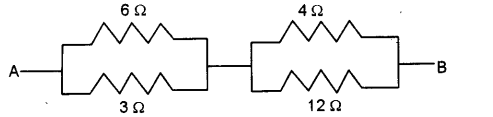(ii) State whether the resistivity of a wire changes with the change in the thickness of the wire.
(c) An electric iron is rated 220 V, 2 kW. 
(i) If the iron is used for 2 h daily find the cost of running it for one week if it costs ₹ 4.25 per kWh.
(ii) Why is the fuse absolutely necessary in a power circuit?
(i) The current which will pass through the electrical device will be I = $$\frac { P }{ V }$$ = $$\frac { 5000 }{ 200 }$$ = 25A
The fuse cannot be used as it will blow off when the current exceeds 8 A.
(ii) Fuse, MCB

(b) (i) 6 Q and 3 Ω are in parallel therefore,
$$\frac{6 \times 3}{6+3}$$ =  $$\frac{18}{9}$$= 2Ω
4 Ω and 12 Ω are in parallel therefore,
$$\frac{4 \times 12}{4+12}$$ = $$\frac{48}{16}$$ = 3Ω
The above two are now in series therefore, R = 2 + 3 = 5
(ii) Resistivity is independent of the dimensions of the wire. Therefore, no change,

(c) (i) V = 220 V, P = 2 kW = 2000 W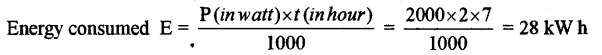Hence cost = 28 x 4.25 = ₹ 119
(ii) A fuse blows off when the maximum current in the circuit is exceeded. This helps in saving the devices connected in the circuit.

Question 9.
(i) Heat supplied to a solid change it into liquid. What is this change in phase called ? 
(ii) During the phase change does the average kinetic energy of the molecules of the substance increase?
(iii) What is the energy absorbed during the phase change called?
(b) (i) State two differences between “Heat Capacity” and “Specific Heat Capacity”. 
(ii) Give a mathematical relation between Heat Capacity and Specific Heat Capacity.
(c) The temperature of 170 g of water at 50°C is lowered to 5°C by adding certain amount of ice to it. Find the mass of ice added. Given: Specific heat capacity of water = 4200 J kg-1 °C-1 and Specific latent heat of ice 336000 J kg-1. 
(a) (i) Melting
(ii) Yes
(iii) Heat of fusion.

(b) (i) The two differences are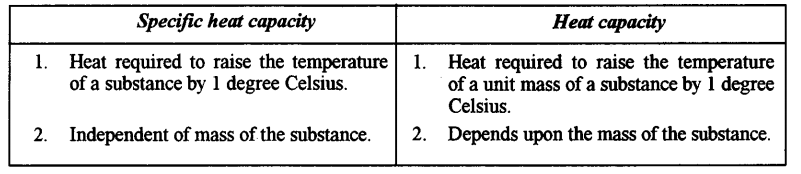(ii) The relation is, heat capacity = specific heat x mass of substance.

(c) Given mass of ice = mg
Mass of water = 170 g
Initial temperature of water = 50 °C
Let the final temperature of the mixture = 5 °C
The latent heat required to change m g of ice at 0 °C to mg of water at 0 °C
= m x 336 = 336 m J
Now heat required to change m g of water at 0 °C to 5 °C
= m x 4.2 x (5 – 0) J = 21m J
Now heat lost by 170 g of water to reach 5 °C from 50 °C
= 170 x 4.2 x (50 – 5) = 32 130 J
Heat lost = Heat gained
336 m + 21 m = 32 130
357 m = 32 130
m = 90 g

Question 10.
(a) The diagram shows a coil wound around a U shape soft iron bar AB.
(i) What is the polarity induced at the ends A and B when the switch is pressed?
(ii) Suggest one way to strengthen the magnetic field in the electromagnet.
(iii) What will be the polarities at A & B if the direction of current is reversed in the circuit?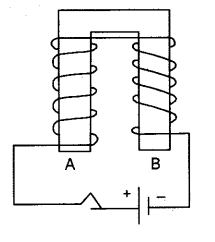(b) The ore of Uranium found in nature contains 92U238 and 92U235. Although both the isotopes are fissionable, it is found out’experimentally that one of the two isotopes is more easily fissionable.
(i) Name the isotope of Uranium which is easily fissionable.
(ii) Give a reason for your, answer.
(iii) Write a nuclear reaction when Uranium 238 emits an alpha particle to form a Thorium (Th) nucleus. 
(c) Radiations given out from a, source when subjected to an electric field in a direction perpendicular to their path are shown below in the diagram. The arrows show the path of the radiation A, B and C. Answer the following questions in terms of A, B and C. 
(i) Name the radiation B which is unaffected by the electrostatic field.
(ii) Why does the radiation C deflect more than A?(iii) Which among the three causes the least biological damage externally?
(iv) Name the radiation which is used in carbon dating.
(i) A north and B south
(ii) Increasing the current through the coil.
(iii)A South and B North

(b) (i) 92u235
(ii) It readily absorbs thermal neutrons and becomes unstable.
(iii) The reaction is 92U23890Th234 + 2He4